# LOOCV (Leave One Out Cross-Validation) in R Programming

LOOCV(Leave One Out Cross-Validation) is a type of cross-validation approach in which each observation is considered as the validation set and the rest (N-1) observations are considered as the training set. In LOOCV, fitting of the model is done and predicting using one observation validation set. Furthermore, repeating this for N times for each observation as the validation set. Model is fitted and the model is used to predict a value for observation. This is a special case of K-fold cross-validation in which the number of folds is the same as the number of observations(K = N). This method helps to reduce Bias and Randomness. The method aims at reducing the Mean-Squared error rate and prevent over fitting. It is very much easy to perform LOOCV in R programming.

#### Mathematical Expression

LOOCV involves one fold per observation i.e each observation by itself plays the role of the validation set. The (N-1) observations play the role of the training set. With least-squares linear, a single model performance cost is the same as a single model. In LOOCV, refitting of the model can be avoided while implementing the LOOCV method. MSE(Mean squared error) is calculated by fitting on the complete dataset.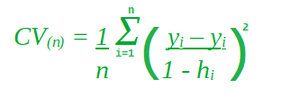In the above formula, hi represents how much influence an observation has on its own fit i.e between 0 and 1 that punishes the residual, as it divides by a small number. It inflates the residual.

#### Implementation in R

The Dataset:

The Hedonic is a dataset of prices of Census Tracts in Boston. It comprises crime rates, the proportion of 25,000 square feet residential lots, the average number of rooms, the proportion of owner units built prior to 1940 etc of total 15 aspects. It comes pre-installed with Eclat package in R.

## R

 `# Installing the package  ` `install.packages``(``"Ecdat"``)  ` `     `  `# Loading package  ` `library``(Ecdat)  ` `     `  `# Structure of dataset in package  ` `str``(Hedonic)`

Output: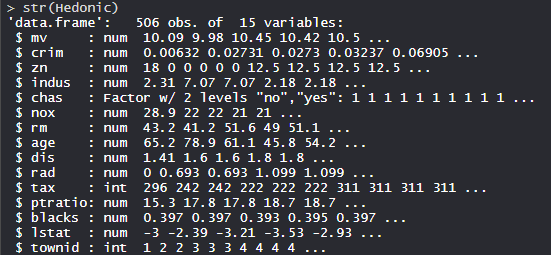Performing Leave One Out Cross Validation(LOOCV) on Dataset:

Using the Leave One Out Cross Validation(LOOCV) on the dataset by training the model using features or variables in the dataset.

## R

 `# Installing Packages ` `install.packages``(``"Ecdat"``) ` `install.packages``(``"boot"``) ` `install.packages``(``"dplyr"``) ` `install.packages``(``"ggplot2"``) ` `install.packages``(``"caret"``) ` ` `  `# Loading the packages ` `library``(Ecdat) ` `library``(boot) ` `library``(dplyr) ` `library``(ggplot2) ` `library``(caret) ` ` `  `# Model Building ` `age.glm <- ``glm``(age ~ mv + crim + zn + indus  ` `               ``+ chas + nox + rm + tax + dis ` `               ``+ rad + ptratio + blacks + lstat, ` `               ``data = Hedonic) ` `age.glm  ` ` `  `# Mean Squared Error ` `cv.mse <- ``cv.glm``(Hedonic, age.glm) ` `cv.mse\$delta ` ` `  `# Generating error of  ` `# Different models ` `cv.mse = ``rep``(0,5) ` `for ``(i ``in` `1:5) ` `{ ` `  ``age.loocv <- ``glm``(age ~ mv + ``poly``(crim, i) ` `                   ``+ zn + indus + chas + nox ` `                   ``+ rm + ``poly``(tax, i) + dis ` `                   ``+ rad + ptratio + blacks ` `                   ``+ lstat, data = Hedonic) ` `  ``cv.mse[i] = ``cv.glm``(Hedonic, age.loocv)\$delta ` `} ` ` `  `cv.mse`

Output:

• Model age.glm: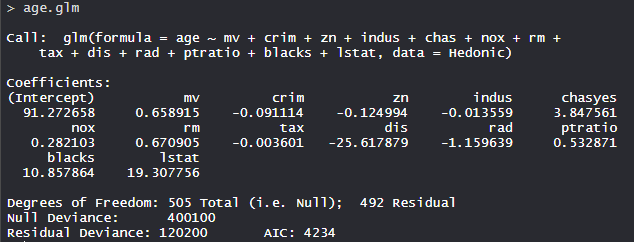The age.glm model has 505 degrees of freedom with Null deviance as 400100 and Residual deviance as 120200. The AIC is 4234.

• Mean Squared Error: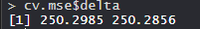The first error 250.2985 is the Mean Squared Error(MSE) for the training set and the second error 250.2856 is for the Leave One Out Cross Validation(LOOCV). The output numbers generated are almost equal.

• Errors of different models: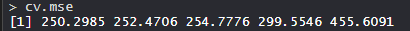The error is increasing continuously. This states that high order polynomials are not beneficial in general case.

#### Advantages of LOOCV are as follows:

• It has no randomness of using some observations for training vs. validation set. In the validation-set method, each observation is considered for both training and validation so it has less variability due to no randomness no matter how many times it runs.
• It has less bias than validation-set method as training-set is of n-1 size. On the entire data set. As a result, there is a reduced over-estimation of test-error as much compared to the validation-set method.

#### Disadvantage of LOOCV is as follows:

• Training the model N times leads to expensive computation time if the dataset is large.

My Personal Notes arrow_drop_upTechnology Enthusiast

If you like GeeksforGeeks and would like to contribute, you can also write an article using contribute.geeksforgeeks.org or mail your article to contribute@geeksforgeeks.org. See your article appearing on the GeeksforGeeks main page and help other Geeks.

Please Improve this article if you find anything incorrect by clicking on the "Improve Article" button below.

Article Tags :

Be the First to upvote.

Please write to us at contribute@geeksforgeeks.org to report any issue with the above content.